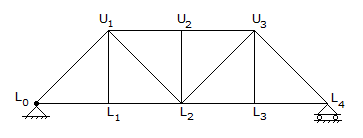# Civil Engineering - UPSC Civil Service Exam Questions

46.

What are the values of tolerable CO concentration in residential area, for continuous exposure durations of 1 hour and 8 hours, respectively ?

 A. 4 and 2 mg / m3 B. 1 and 2 mg / m3 C. 4 and 6 mg / m3 D. 5 and 1 mg / m3

Explanation:

No answer description available for this question. Let us discuss.

47.

If the proportion of soil passing 75 micron sieve is 50% and the liquid limit and plastic limit are 40% and 20% respectively, then the group index of the soil is

 A. 3.8 B. 6.5 C. 38 D. 65

Explanation:

No answer description available for this question. Let us discuss.

48.

Which one of the following instruments can be used as a clinometer ?

 A. Prism square B. Line ranger C. Abney level D. Optical square

Explanation:

No answer description available for this question. Let us discuss.

49.

Consider the following statements :
For the N-girder shown in the given figure, ILD for force in the member, L0U1 is obtained by
1. multiplying the ordinate of ILD for shear in the panel L0L1, by sec θ.
2. dividing the ordinate of ILD for moment at L1 by cos θ x L0L1
3. dividing the ordinate of ILD for moment at L1 by L1 U1
Of these statements :A. only 3 is correct B. 1 and 3 are correct C. 1 and 2 are correct D. 1, 2 and 3 are correct

Explanation:

No answer description available for this question. Let us discuss.

50.

Consider the following statements :
1. In a fluid under motion, the pressure at a point is always perpendicular to the surface.
2. Pascal's law states that the pressure at a point is same in all directions.
3. Magnitude of pressure of a fluid at a point is obtained from hydrostatic law.
4. Pascal's law is applicable in the operation of a hydraulic press.
Which of these statements are correct ?

 A. 1, 2 and 3 B. 1, 3 and 4 C. 1, 2 and 4 D. 2, 3 and 4